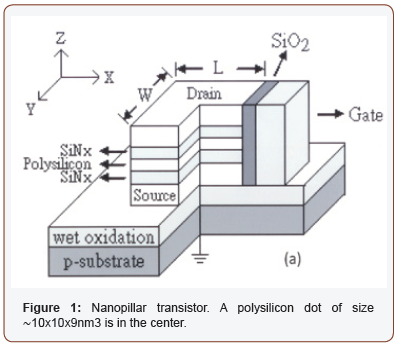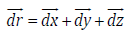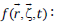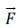# Evidence of Electron Charging Induced Alternating Currents in Nanopillar Transistor

Te Chien Wang and Yue Min Wan*

Department of Electronic Engineering, I-Shou University, Taiwan

Received Date: June 06 , 2019;  Published Date: June 10 , 2019

#### Abstract

Study of electron charging in nanopillar transistor at 300K shows that elastic vibration is an automatic behavior in the device. The frequency observed in the drain-source current is found to agree with the charging frequency. Given a quantum dot of size ~10x10x9nm3, the maximum displacement estimated is 0.3nm. Once the displacement diminishes to zero, single-electron tunnel dominates the I-V. A forced vibration model is proposed to explain the correlation between surface charges and vibrations. When the distribution of charges is uniformly on each SiNx atom, vibration becomes stable and can yield homogenous damping current.

Keywords:Quantum dot; Nanopillar transistor; Pulse voltage; Mechanical vibration

#### Introduction

Electron transport in electro-mechanical quantum dot transistors  has been a hot topic for more than three decades. No doubt it is very interesting for engineers as it has the potential to revolutionize semiconductor technology. The disclosure of single electron tunnel is definitely a hall mark effect because it can transform into the useful devices such as single-electron pump , single-electron memory cell  and single-electron detectors or counters . Along with these works, we have presented “nanopillars” transistor . In this device, the structure is in vertical form; drain current versus drain-source voltage measurements show excellent feature of single-electron charging. By matching the charging peaks with the parallel plate charging energy Ec = e2/2C, single electron effect is evident.

However, a critical issue remains unanswered is that the onset of peaks is not well defined. Early studies at low temperatures  found similar effect and it was attributed to the so-called “Coulomb blockade” where the first electron is blocked by Coulomb energy and no electrical current is allowed to move. But recently, quantum shuttle  was proposed to deliberate that at finite temperatures electrons could move from one electrode to another electrode via the repetitive motion of the central quantum dot. Consequently, current noises  appear. In this highly unstable region, mechanisms of mechanical dissipation , elastic deformation and electro-mechanical feedback  can stand out and play vital roles. As such, it definitely worth to do carefully experiments in this region. As expected, we find that at low charging voltages, the I-V does show drastically different behavior. What observed are giant current oscillations from the very beginning. After reaching a peak the current decreases quickly to zero. To understand these effects, a forced vibration model is proposed to explain the data and good agreements are found.

#### Device

The transistor, schematically drawn in Figure 1, was fabricated on p-type (100) silicon wafers. It basically features a center silicon separated from the top and bottom contacts by two nitride barriers. This dot cavity has a critical length of 3 nm and is intimately coupled to a gate electrode on the side. The device was then loaded into a probe station (Thermal Cascade) for current-voltage measurements. HP 4156 C three-terminal meter was used. It had the resolution of 1mV and 10 fA. Since our model is intimately related to the manner of electrical charging, in Figure 2(a), the pulsed voltages are schematically drawn. Within the time period of 1mS, 1mV was first applied on the first half cycle. After that another 1mV was added to the 1mV for a total of 2mV on the device in the next period.#### Theoretical Model

This kind of charging is commonly known by experimentalists as it can avoid overheating. In Figure 2(b), when certain amounts of charges Qs are accumulated on the surface of the bottom nitride layer, it will create a force to bend the layers. On the other end of the layers, a positive current +I(t) is produced. The elasticity of all layers, here marked as energy ΔE, then will force a backward movement moment later to create a negative current –I(t). As a consequence, alternating currents appear. Correspondingly, displacement x(t) will change between +α and –α as illustrated in Figure 2(c).The best model for such behavior is the dynamical equation 𝑚ẍ(t)+cẋ(t)+kx(t)=f(t) , where ẍ(t) is the second order time derivative of x(t); ẋ(t) is the first order derivative; x(t) is the displacement; m represents the mass of the system as shown in Figure 3, c denotes the damping coefficient, k is the spring constant and f(t) is the applied force.

To solve this equation, two conditions must be considered. One is the zero-force f(t)=0 case and the other is the finite force case, f(t)>0. The solution xg(𝑡) is a textbook example and it iswhereα is the maximum displacement, 𝜉 is the ratiois the nature frequency. Inside the bracket of the sinusoidal functionresponse frequency. With this identity, one can calculate the current. Note that Ig(t)= neAυd where n is the volume density of electrons, A is layer area, υd is the drift velocity as defined by ẋ(t). Given all the parameters, the current created by the damping vibration is found to beOther than the homogeneous current of Ig(t), there is the particular current Ip(t). Derivation of it is different from the Ig(t) and that it is discussed in reference . Once the theoretical derivation is finished, the data is fitted and compared.

#### Data Analysis

In Figure 4(a), a Ig(t) curve is plotted in the window of 0 – 0.25 s for comparison to the data. This result is obtained by several tries on the ω and the ξ which appear to be the most critical parameters in the model. Notice that these alternating current mimics the main features of the data in the bottom figure very well. In Figure 4(b), the good fitting comes from the range between 0 and 0.17 V where a smaller damping and a slightly higher ω appears to dominate the whole spectrum. After the ac damping, the transistor becomes stable and single electron tunnel appears and dominates the transport. This analysis answers the key query of this paper and also proves that mechanical stability is an essential part for the upcoming quantum tunnel of electrons.None.

#### Conflict of Interest

No conflict of interest.

Article Details
Citation
Keywords
Scroll to
Scroll to Top